# Quiz 14: Determining Project Progress and Results

Business

Presenting valuable decision-making data to the multiple resources and leadership groups required to support a project provides the critical linkage between the feedback of law data and ability to successful control a single project or the entire multiple portfolio. Project data collection and management presents the opportunity to simultaneously manage an organization's "profit, people, and planet" objectives in an optimal way. The fundamental reason for determining project progress and results comes down to one thing - Presenting actionable, decision-making information to project leaders.

If project progress is not planned then possible changes may occur. For example, certainly making adjustments to schedule or budget are possibilities. Also, scope may need to be adjusted. More resources may need to be acquired. Therefore possible changes is necessary for project progress.

By extracting the information :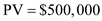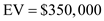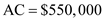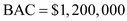Part (a) Calculate the scheduled variance (SV) Methodology: The formula for calculating scheduled variance is given below: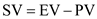Where, The scheduled variance is SV The earned value is EV The planned value is PV By substituting : The earned value is 350,000 and the planned value is 500,000.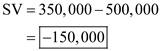Therefore, it can be concluded that scheduled value is a negative value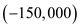, illustrating that the project is behind schedule, and the earned value is less than the actual cost. Part (b) Calculate the cost variance (CV) Methodology: The formula for calculating cost variance is given below: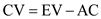Where, The cost variance is CV The earned value is EV The actual cost is AC By substituting : The earned value is 350,000 and the actual cost is 550,000.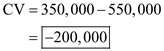Therefore, it can be concluded that cost variance is a negative value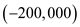, illustrating that the project is over budget, and the earned value is less than the actual cost. Part (c) Calculate the scheduled performance index (SPI) Methodology : The formula for calculating the scheduled performance index is given below: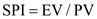Where, The scheduled performance index is SPI The earned value is EV The planned value is PV By substituting : The earned value is 350,000 and the planned value is 500,000.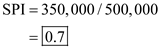Therefore, it can be concluded that the scheduled performance index has a value less than 1, so the project is behind schedule. Part (d) Calculate the cost performance index (CPI) Methodology: The formula for calculating the cost performance index is given below: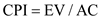Where, The cost performance index is CPI The earned value is EV The actual cost is AC By substituting : The earned value is 350,000 and the actual cost is 550,000.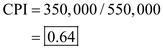Therefore, it can be concluded that the cost performance index has a value less than 1, so the project is over budget. Part (e) Calculate the estimate to complete (ETC) Methodology: The formula for calculating estimate to complete is given below: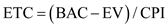Where, The estimate to complete is ETC The earned value is EV The cost performance index is CPI The budget at completion is BAC By substituting : The earned value is 350,000, the budget at completion is 1,200,000, and the cost performance index is 0.64.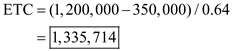Therefore, it can be concluded that the project value exceeds the budget at completion by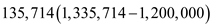. Part (f) Calculate the estimate at completion (EAC) Methodology: The formula for calculating the estimate at completion is as follows: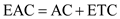Where, The estimate at completion is EAC The estimate to complete is ETC The actual cost is AC By substituting : The estimate to complete is 1,335,714 and the actual cost is 550,000.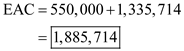Therefore, it can be concluded that the estimate at completion is over budget. Conclusion: Therefore, the conclusion can be drawn that the manager of the project should conduct a meeting with all the stakeholders of the company to determine how to proceed. The manager of the project should investigate why the project is behind schedule or over budget.

There is no answer for this question

There is no answer for this question

There is no answer for this question